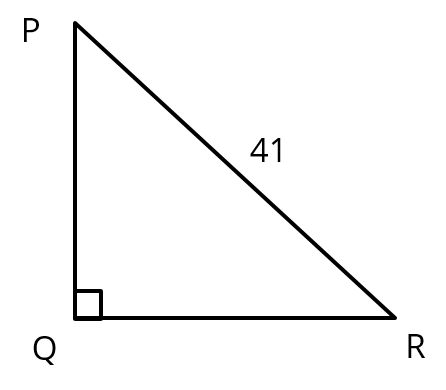# Trigonometry Important Question | Class 10 Maths | Question 6

###### CBSE Class 10 Chapter 8 | Trigonometric Values & Quadratic EquationsClass 10 Math Questions

This CBSE class 10 Maths extra question is from the topic Trigonometry. Concept Covered: Calculating trigonometric values and using quadratic equations & Pythagoras theorem.

Question 6 : In triangle PQR, right angled at Q if PR = 41 units and PQ – QR = 31. Find sec2R – tan2R.

## NCERT Solution to Class 10 Maths

### Explanatory Answer | Trigonometry Extra Question 6

PQR with right angled at Q
PR = 41 units
PQ - QR = 31
PQ - QR = 31 → PQ = 31 + QR#### Step 1: Compute QR and PQ

Using Pythagoras Theorem, PR2 = PQ2 + QR2
412 = (31 + QR)2 + QR2
1681 = 961 + 62QR + QR2 + QR2
2QR2 + 62QR – 720 = 0
Divide the equation by 2: QR2 + 31QR – 360 = 0

Factorize QR2 + 31QR – 360 = 0 QR2 + 40 QR – 9QR – 360 = 0
QR(QR + 40) – 9(QR + 40) = 0
(QR + 40) (QR – 9) = 0
QR = -40 or QR = 9
QR cannot be negative ∴ QR = 9
PQ = QR + 31 = 9 + 21 = 40

#### Step 2: Calculate sec2R – tan2R

sec R = $$frac{\text{hypotenuse}}{\text{side adjacent to ∠R}}$ =$\frac{\text{PR}}{\text{QR}}$= $\frac{\text{41}}{\text{9}}$ tan R = $\frac{\text{side opposite to ∠R}}{\text{side adjacent to ∠R}}$ = $\frac{\text{40}}{\text{9}}$ sec2R – tan2R =$\frac{41^2}{9^2}$–$\frac{40^2}{9^2}$ $\frac{\text{$41 + 40$} × $text{$41 - 40)}}{$text{81}}$ =$\frac{\text{81}}{\text{81}}$ = 1

So, sec2R – tan2R = 1

###### Free CBSE Online CoachingClass 10 Maths

Register in 2 easy steps and
Start learning in 5 minutes!